Warning

# Dynamic programming

This assignment will focus on the discrete optimization technique called dynamic programming. The main idea behind the dynamic programming is subdivision of a complex problem to several simpler subproblems, solving these subproblems separately and combining solutions of the subproblems to obtain a solution of the original complex problem. Each individual subproblem is solved only once and its solution is cached to avoid solving the subproblem repeatedly.

## Part 1

### Image path

Let us consider paths in an image which conform to the following specification. A path goes from the top image row to the bottom image row. It contains exactly one pixel from each row of the image. Finally, when going from one image row to the next, the path can either continue into the same column, or go to one of the neighboring columns (left or right one). Formally, the valid path for an image of size $M \times N$ ($M$ is height, $N$ is width) consists of pixels $((1,j_1),(2,j_2),\ldots,(M,j_M))$ where $1 \leq j_i \leq N$ for $i = 1 \ldots M$ and $|j_{i+1}-j_i| \leq 1$ for $i = 1 \ldots M-1$.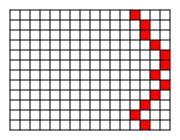Figure 1. Example of a valid downward path.

### Graph representation of images

We represent the image as a graph. Vertices of the graph correspond to individual pixels of the image. There are edges connecting vertices from two neighboring rows if the vertices are located in the same column, one column to left or one column to right. Formally, the image is represented by the graph $G=(V,E)$ where $V = \{ v_{i,j} : i = 1 \ldots M, j = 1 \ldots N \}$ and $E = E_{\mathrm{TL}} \cup E_{\mathrm{T}} \cup E_{\mathrm{TR}}$ where: \begin{align*} E_{\mathrm{TL}} &= \{ (v_{i-1,j-1},v_{i,j}) : i = 2 \ldots M, j = 2 \ldots N \} \\ E_{\mathrm{T}} &= \{ (v_{i-1,j},v_{i,j}) : i = 2 \ldots M, j = 1 \ldots N \} \\ E_{\mathrm{TR}} &= \{ (v_{i-1,j+1},v_{i,j}) : i = 2 \ldots M, j = 1 \ldots N-1 \} \end{align*} The edges $E_{\mathrm{TL}}$ connect vertices in one row with vertices located in top-left direction in the previous row, $E_{\mathrm{T}}$ in top direction and $E_{\mathrm{TR}}$ in top-right direction.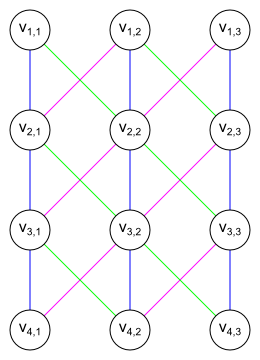$\bullet$$E_{\mathrm{TL}} \bullet$$E_{\mathrm{T}}$ \bullet$$E_{\mathrm{TR}} Figure 2. Sample graph representing 4 \times 3 image. We are interested in vertical paths which contain exactly one vertex from each row. Formally, a valid path is P = ( v_{1,j_1}, (v_{1,j_1},v_{2,j_2}), v_{2,j_2}, \ldots, (v_{M-1,j_{M-1}},v_{M,j_M}), v_{M,j_M} ). The structure of the graph ensures that paths do not skip more than one column while going from one row to the row below.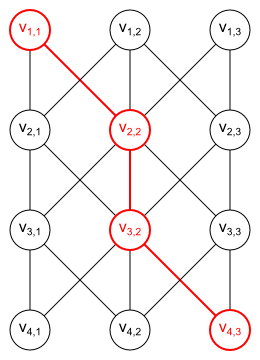Figure 3. Example of a path ( v_{1,1}, (v_{1,1},v_{2,2}), v_{2,2}, (v_{2,2},v_{3,2},), v_{3,2}, (v_{3,2},v_{4,3}), v_{4,3} ). ### Shortest vertical paths using dynamic programming - example Let us now extend our graph by assigning weights (costs) to its vertices and edges. Since the graph represents some image, the costs are based on features computed from the image. The way of computing the costs depends on the task which we want to solve. We will later show several interesting problems which can be solved in the proposed framework. For now, let us assume that the costs have been given. Let the costs of vertices and edges in a graph be denoted as specified here: Entity Cost vertex v_{i,j} C_{\mathrm{V}}(i,j) top-left edge (v_{i-1,j-1},v_{i,j}) \in E_{\mathrm{TL}} C_{\mathrm{TL}}(i,j) top edge (v_{i-1,j},v_{i,j}) \in E_{\mathrm{T}} C_{\mathrm{T}}(i,j) top-right edge (v_{i-1,j+1},v_{i,j}) \in E_{\mathrm{TR}} C_{\mathrm{TR}}(i,j)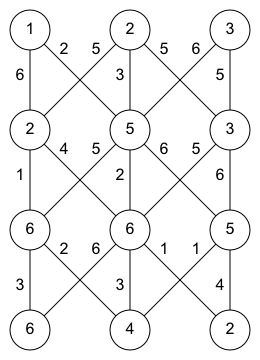C_{\mathrm{V}} 1 2 3 1 1 2 3 2 2 5 3 3 6 6 5 4 6 4 2 C_{\mathrm{TL}} 1 2 3 1 X X X 2 X 2 5 3 X 4 6 4 X 2 1 C_{\mathrm{T}} 1 2 3 1 X X X 2 6 3 5 3 1 2 6 4 3 3 4 C_{\mathrm{TR}} 1 2 3 1 X X X 2 5 6 X 3 5 5 X 4 6 1 X Figure 3. Example vertex and edge costs. For each vertex v_{i,j}, we want to find the shortest path starting in some vertex from the first row and ending in v_{i,j}. We denote such shortest path P_{i,j} and its cost C_{\mathrm{P}}(i,j). It is equal to the sum of costs of all vertices and edges on the path. The shortest paths can be found incrementally using the dynamic programming approach. In i-th step, the shortest paths P_{i,1} \ldots P_{i,N} to all vertices of the i-th row v_{i,1} \ldots v_{i,N} are computed using the previously computed shortest paths P_{i-1,1} \ldots P_{i-1,N} to all vertices of the previous row i-1. For each vertex v_{i,j} where i > 1, it is convenient to remember the column index of the vertex preceding v_{i,j} on the shortest path P_{i,j}. It will help us to reconstruct the shortest path. Let us denote such index D(i,j) for i > 1. Since only top-left, top or top-right edge can be used to move to the vertex v_{i,j}, it always holds D(i,j) \in \{j-1,j,j+1\}. Let us use our sample graph to explain the dynamic programming algorithm computing C_{\mathrm{P}}(i,j) and D(i,j) incrementally from row to row. #### Row 2 Let us assume that we have been already given the shortest paths P_{2,1}, P_{2,2}, P_{2,3} to the vertices of the second row, as shown in the figure below. The values plot in the vertices of the second row denote the shortest path costs C_{\mathrm{P}}(2,1) = 9, C_{\mathrm{P}}(2,2) = 8, C_{\mathrm{P}}(2,3) = 10. The shortest paths themselves are plotted in red. They are determined by the column indices of the preceding vertices D(2,1) = 1, D(2,2) = 1, D(2,3) = 2.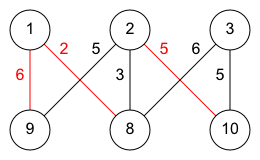C_{\mathrm{P}} 1 2 3 1 1 2 3 2 9 8 10 D 1 2 3 1 X X X 2 1 1 2 Figure 4. Shortest path costs and indices of preceding vertices for the second row of the graph. #### Row 3 Now we want to construct the shortest path P_{3,1} to the vertex v_{3,1}. There are two candidates for it: 1. We can either use the shortest path P_{2,1} to the vertex v_{2,1} and then use the top edge (v_{2,1}, v_{3,1}). It is plotted blue in the figure below. The cost of such path would be:$$C_{\mathrm{P}}(2,1) + C_{\mathrm{T}}(3,1) + C_{\mathrm{V}}(3,1) = 9 + 1 + 6 = 16$$2. Or we can use the shortest path P_{2,2} to the vertex v_{2,2} and then use the top-right edge (v_{2,2}, v_{3,1}). It is plot cyan in the figure below. The cost of such path would be:$$C_{\mathrm{P}}(2,2) + C_{\mathrm{TR}}(3,1) + C_{\mathrm{V}}(3,1) = 8 + 5 + 6 = 19$$The first option gives the lower cost and so P_{3,1} = P_{2,1} \oplus ( (v_{2,1}, v_{3,1}), v_{3,1} ) where \oplus denotes a concatenation. The cost of the path is C_{\mathrm{P}}(2,1) = 16. The vertex v_{2,1} is preceding the vertex v_{3,1} on the shortest path and thus D(3,1) = 1.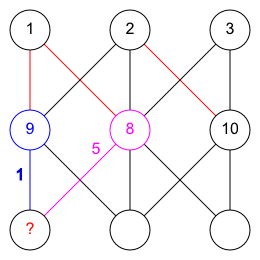Figure 5. Constructing the path P_{3,1}. The shortest path P_{3,2} to the vertex v_{3,2} can be constructed analogously. There are three candidates: 1. Use the path P_{2,1} and the top-left edge (v_{2,1}, v_{3,2}), having the cost 9 + 4 + 6 = 19. 2. Use the path P_{2,2} and the top edge (v_{2,2}, v_{3,2}), having the cost 8 + 2 + 6 = 16. 3. Use the path P_{2,3} and the top-right edge (v_{2,3}, v_{3,2}), having the cost 10 + 5 + 6 = 21. The second option gives the minimal cost. Thus C_{\mathrm{P}}(3,2) = 16 and D(3,2) = 2.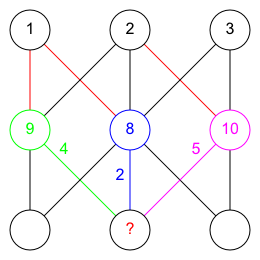Figure 6. Constructing the path P_{3,2}. The shortest path P_{3,3} is computed analogously.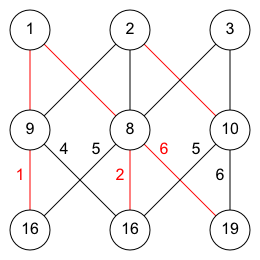C_{\mathrm{P}} 1 2 3 1 1 2 3 2 9 8 10 3 16 16 19 D 1 2 3 1 X X X 2 1 1 2 2 1 2 2 Figure 7. Costs of the shortest paths and indices of the preceding vertices for the third row of the graph. #### Row 4 Finally, the following figure shows the shortest path costs for all vertices of the graph.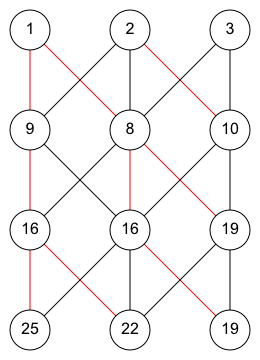C_{\mathrm{P}} 1 2 3 1 1 2 3 2 9 8 10 3 16 16 19 4 25 22 19 D 1 2 3 1 X X X 2 1 1 2 3 1 2 2 4 1 1 2 Figure 8. Costs of the shortest paths and indices of the preceding vertices for all vertices in our sample graph. #### Selection of the minimal cost path The next step is to select the vertex from the last row having the minimal path cost. There are three vertices in the last row v_{4,1}, v_{4,2}, v_{4,3} having path costs C_{\mathrm{P}}(4,1) = 25, C_{\mathrm{P}}(4,2) = 22, C_{\mathrm{P}}(4,3) = 19. The path P_{4,3} is therefore the shortest vertical path going from the first row to the last row. #### Reconstruction of the shortest path The shortest path P_{4,3} can be reconstructed recursively, using the computed table of indices D(i,j). Remember that D(i,j) is for each vertex v_{i,j} equal to the column index of the vertex preceding the vertex v_{i,j} on the shortest path P_{i,j}. Since D(4,3) = \color{red}{2}, v_{3,\color{red}{2}} precedes v_{4,3} on P_{4,3}. Recursively, since D(3,2) = \color{red}{2}, v_{2,\color{red}{2}} precedes v_{3,2} on P_{3,2} and P_{4,3}. Finally, since D(2,2) = \color{red}{1}, v_{1,\color{red}{1}} is the first vertex of P_{4,3}.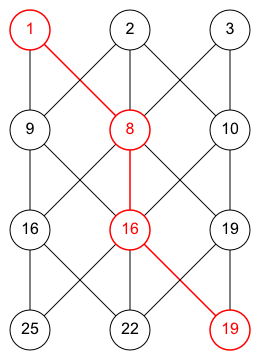D 1 2 3 1 X X X 2 1 \color{red}{1} 2 3 1 \color{red}{2} 2 4 1 1 \color{red}{2} Figure 9. The shortest path P_{4,3} is reconstructed using the precomputed table D(i,j) by tracing the preceding vertices recursively. ### Shortest vertical paths using dynamic programming - implementation Implement the algorithm finding the shortest vertical path in MxN grid-like graph using the described dynamic programming approach. Split the implementation to the following functions: • [path_cost, path_idx] = dp_path_optim(vertex_cost, topleft_cost, top_cost, topright_cost): For each vertex v_{i,j} compute its shortest path cost and column index to the previous vertex on the shortest path. All the inputs and outputs are MxN matrices. • path_cost(i,j) = C_\mathrm{P}(i,j) • path_idx(i,j) = D(i,j) for i > 1; path_idx(1,:) can be arbitrary as there are no vertices preceding vertices from the first row • vertex_cost(i,j) = C_\mathrm{V}(i,j) • topleft_cost(i,j) = C_\mathrm{TL}(i,j) for j > 1; topleft_cost(:,1) is not well defined as there are no top-left edges going to the first column vertices • top_cost(i,j) = C_\mathrm{T}(i,j) for i > 1; top_cost(1,:) is not well defined as there are no top edges going to the row • topright_cost(i,j) = C_\mathrm{TR}(i,j) for j < N; topright_cost(:,N) is not well defined as there are no top-right edges going to the last column vertices • path = dp_path_trace(path_cost, path_idx): Trace the shortest vertical path going from the first to the last row of M \times N grid-like graph. • path is Mx1 vector such that path(i) = j if the vertex v_{i,j} lies on the shortest vertical path • path_cost(i,j) = C_\mathrm{P}(i,j) • path_idx(i,j) = D(i,j) for i > 1; path_idx(1,:) is not well defined as there are no vertices preceding vertices from the first row • [path, path_cost, path_idx] = dp_path(vertex_cost, topleft_cost, top_cost, topright_cost): Combine the functions dp_path_optim and dp_path_trace. • path is Mx1 vector such that path(i) = j if the vertex v_{i,j} lies on the shortest vertical path • path_cost(i,j) = C_\mathrm{P}(i,j) • path_idx(i,j) = D(i,j) for i > 1; path_idx(1,:) can be arbitrary as there are no vertices preceding vertices from the first row • vertex_cost(i,j) = C_\mathrm{V}(i,j) • topleft_cost(i,j) = C_\mathrm{TL}(i,j) for j > 1; topleft_cost(:,1) is not well defined as there are no top-left edges going to the first column vertices; this parameter is optional and if it is not defined then zero costs are used by default • top_cost(i,j) = C_\mathrm{T}(i,j) for i > 1; top_cost(1,:) is not well defined as there are no top edges going to the row; this parameter is optional and if it is not defined then zero costs are used by default • topright_cost(i,j) = C_\mathrm{TR}(i,j) for j < N; topright_cost(:,N) is not well defined as there are no top-right edges going to the last column vertices; this parameter is optional and if it is not defined then zero costs are used by default Start your implementation with downloading the following package. It contains the headers and documentation of the functions above which you need to complete. Implementation notes: The function dp_path_optim iteratively computes path_cost(i,:) and path_idx(i,:) for i > 1. Since Matlab performance in for-loops is very limited, you should compute path_cost(i,:) and path_idx(i,:) for whole row at once, i.e. not using for-loop to go through individual columns. The minimization can be performed in one step using the following 3 \times N matrix:  \infty C_{\mathrm{P}}(i-1,1) + C_{\mathrm{TL}}(i,2) \ldots C_{\mathrm{P}}(i-1,N-2) + C_{\mathrm{TL}}(i,N-1) C_{\mathrm{P}}(i-1,N-1) + C_{\mathrm{TL}}(i,N) C_{\mathrm{P}}(i-1,1) + C_{\mathrm{T}}(i,1) C_{\mathrm{P}}(i-1,2) + C_{\mathrm{T}}(i,2) \ldots C_{\mathrm{P}}(i-1,N-1) + C_{\mathrm{T}}(i,N-1) C_{\mathrm{P}}(i-1,N) + C_{\mathrm{T}}(i,N) C_{\mathrm{P}}(i-1,2) + C_{\mathrm{TR}}(i,1) C_{\mathrm{P}}(i-1,3) + C_{\mathrm{TR}}(i,2) \ldots C_{\mathrm{P}}(i-1,N) + C_{\mathrm{TR}}(i,N-1) \infty The infinite values code a missing top-left edge for the vertex v_{i,1} and a missing top-right edge for the vertex v_{i,N}. In Matlab, the matrix can be constructed as: [Inf, path_cost(i-1,1:N-1) + topleft_cost(i,2:N); path_cost(i-1,:) + top_cost(i,:); path_cost(i-1,2:N) + topright_cost(i,1:N-1), Inf] If the inbuilt Matlab function min is applied on such matrix, it returns two parameters: 1) 1xN vector of minimum values for each column which can be used as path_cost(i,:) after certain modification; 2) 1xN vector of the row indices where the minimum is located for each column which which can be used as path_idx(i,:) after certain modification. ### Tracing vessel in image A simple application of the described dynamic programming algorithm is tracing of the vertical vessel in the following medical image. We want to transform the problem of tracing the vertical vessel in the image to the problem of finding the shortest vertical path in the graph. Note that the pixels corresponding to the vessel are the lightest ones, while the background is rather dark. Based on this observation, we set the costs of the vertices corresponding to the light pixels lower than costs of the dark pixel vertices. Specifically, we use the vertex cost C_{\mathrm{V}}(i,j) = 1 - I(i,j) where I(i,j) \in [0, 1] is the intensity of the pixel (i,j) (intensity 0 for black, 1 for white). The edge costs C_{\mathrm{TL}}, C_{\mathrm{T}}, C_{\mathrm{TR}} can be set to zeros, as the intensity information stored in the vertices is sufficient to trace the vessel correctly. You can use the the script hw_dynprog_1.m to load the image, display all costs and the traced vessel. Note that the path found by your algorithm does not need to match the path shown in the image above exactly, because there can be more paths having the same (minimal) cost. ## Part 2 ### Seam carving The described algorithm for searching the shortest vertical paths can also be used for content aware image resizing, also known as seam carving. The primary goal is to automatically reduce width or height of the image by deleting some of its pixels, while preserving its most important contents. Additionally, the user is allowed to select the objects in the image which should be certainly deleted and the objects which should be certainly protected from being removed. The algorithm is based on incremental construction of vertical or horizontal seams which are being removed from the image. The following image shows a person watching a tower. The person and the tower form obviously the main contents of the image. Let us suppose that we want to decrease the width of the image by 200 pixels. The next figure shows an example of a vertical seam going through not so important background. The seam corresponds to vertical path in the image, i.e. it contains exactly one pixel from each row of the image and between two following rows it stays in the same column or it skips one column to left or it skips one column to right. If the seam is removed from the image, the image width is decreased by one. The procedure of finding a vertical seam and removing it from the image can be repeated several times. The image width is reduced by one in each iteration. The reduced image is the input of the next iteration. The next figure shows 200 incrementally constructed vertical seams. They were all projected to the original image for the purpose of visualization. Note that none of the seams is going through the person or the tower. The following image is the output of the seam carving algorithm after 200 iterations. Its width is 200 pixels less than the original one. The extended seam carving algorithm makes it possible for the user to select objects in the image which should be certainly deleted by seam carving and objects to be preserved. The selection is achieved by labeling the pixels through which the seams should pass (plotted in transparent red) and pixels which all seams should avoid (plotted in transparent blue). The following image shows the carved seams. The number of seams was chosen so that the whole object marked for deletion is removed, i.e. the number of seams is equal to width of the marked area. Finally, this is the result obtained by incremental removal of seams. ### Standard seam carving We formulate the problem of finding the vertical seam avoiding the important image contents in our framework of finding the shortest vertical path in the graph. Vertices of the graph correspond to M \times N image pixels. There are several options of setting the vertex costs. The reasonable heuristics is summation of the absolute values of the partial derivatives of the image in x and y direction:$$C_{\mathrm{V}}(i,j) = \left| \frac{\partial I}{\partial x}(i,j) \right| + \left| \frac{\partial I}{\partial y}(i,j) \right|Here I denotes the grayscale image (M \times N matrix in Matlab) obtained from the original RGB image (M \times N \times 3 matrix). Use Matlab function rgb2gray to convert the RGB image to the grayscale image. If the pixel having low absolute value of the partial derivatives is removed, its neighboring pixels usually blend together easily without causing any visible artifacts. Moreover, the object boundaries having high absolute partial derivatives are avoided by the seams. We will estimate the partial derivatives by convolving the image with the vertical and horizontal version of scaled Sobel operator: \begin{align*} \frac{\partial I}{\partial x} =& I * \begin{bmatrix}\frac{1}{8} & 0 & -\frac{1}{8}\\\frac{1}{4} & 0 & -\frac{1}{4}\\\frac{1}{8} & 0 & -\frac{1}{8}\end{bmatrix}\\ \frac{\partial I}{\partial y} =& I * \begin{bmatrix}\frac{1}{8} & \frac{1}{4} & \frac{1}{8}\\0 & 0 & 0\\-\frac{1}{8} & -\frac{1}{4} & -\frac{1}{8}\end{bmatrix} \end{align*} There is a function called conv2 in Matlab which accepts M \times N matrix of pixel intensities and 2D convolution kernel (having size 3 \times 3 in case of Sobel operator) as its arguments (use 'same' for the shape parameter). The edge costs C_{\mathrm{TL}}, C_{\mathrm{T}}, C_{\mathrm{TR}} are set to zeros, as the vertex costs are sufficient to find plausible seam. The following figure shows vertex costs computed for the tower image: ### Forward seam carving The forward seam carving algorithm is similar to the standard seam carving. The only difference is in using another set of vertex and edge costs. The vertex costs C_{\mathrm{V}} are set to zeros. The edge costs express the differences between pixels which are not currently direct neighbors (in sense of 4-connected neighborhood of pixels), but they would become neighbors after carving a certain pixel from their neighborhood. The following image shows three possible cases of carving the seam along top-left edge, top edge or top-right edge coming to the pixel (i,j). The pixels plotted in red are carved out from 2 \times 3 group of pixels. The carving operation results in 2 \times 2 group of pixels. Carving the red pixels out causes the pixels, which have not been neighbors previously, to become neighbors. The borders between the newly originated pairs of neighbors are plot in red. There are two new pairs of neighbors for the top-left edge: \{(i,j+1), (i,j-1)\} and \{(i-1,j), (i,j-1)\}. There is only one new pair for the top edge: \{(i,j-1), (i,j+1)\}. Note that \{(i-1,j-1),(i-1,j+1)\} do not form a new pair because they are considered in the previous row i-1. Finally, there are two pairs of neighbors for the top-right edge: \{(i,j-1), (i,j+1)\} and \{(i-1,j), (i,j+1)\}. Based on the image above, we define for i = 2 \ldots M the edge costs as follows: \begin{align*} C_{\mathrm{TL}}(i,j) &= \begin{cases} ||I(i,j+1) - I(i,j-1)|| + ||I(i-1,j) - I(i,j-1)||, \hspace{5pt}\text{ where } j \in \{2 \ldots N-1\}\\ \infty, \hspace{5pt}\text{ where } j=N \end{cases}\\ C_{\mathrm{T}}(i,j) &= \begin{cases} ||I(i,j+1) - I(i,j-1)||, \hspace{5pt}\text{ where } j \in \{2 \ldots N-1\}\\ \infty, \hspace{5pt}\text{ where } j \in \{1,N\} \end{cases}\\ C_{\mathrm{TR}}(i,j) &= \begin{cases} ||I(i,j+1) - I(i,j-1)|| + ||I(i-1,j) - I(i,j+1)||, \hspace{5pt}\text{ where } j \in \{2 \ldots N-1\}\\ \infty, \hspace{5pt}\text{ where } j=1 \end{cases}\\ \end{align*} Here I is RGB image of size M \times N, i.e. I(i,j) \in [0,1]^3. The function ||\cdot|| denotes the standard Euclidean norm. The edge costs are therefore based on the differences between RGB values of the neighboring pixels. Note that the costs of top-left and top-right edges sum distance for two pairs of pixels, while the top edge cost is only based on one pair. This makes the top edge costs in general lower than the top-left and top-right costs. The top edges are therefore slightly preferred. Also note that costs for the first/last column are set to \infty, because the border pixels have no left/right neighbor and therefore it is not possible to compute the required distances. Implementation note: It is not possible to copy and paste the above equations to Matlab directly. If img is M \times N \times 3 matrix representing RGB image then img(i,j) gives only the value of the red channel at position (i, j). You need to use img(i,j,:) to get the full RGB vector represented as 1 \times 1 \times 3 matrix. You may find the in-built function squeeze usefull to get rid of singleton dimensions. ### Pixels deletion and protection It is possible to modify vertex costs C_{\mathrm{v}} of certain pixels to new costs C_{\mathrm{v}}^{'} so that the seam is forced to avoid them or conversely go through them. The costs of the other pixels remain unchanged. To avoid certain pixel (i,j) from being deleted, its vertex cost C_{\mathrm{v}}^{'}(i,j) is set to a very high value. Then each path containing the vertex v_{i,j} has very high cost and therefore such path can never be selected as the shortest path. Therefore, such path never becomes a seam to be carved. To force certain pixel (i,j) to be part of the carved seam, the corresponding vertex cost C_{\mathrm{v}}^{'}(i,j) is set to a very low value. Then any path containing the vertex v_{i,j} has lower cost than each path not containing v_{i,j}. Therefore, some path containing v_{i,j} is chosen as a seam to be carved. It remains to determine the high and low values of costs for pixels to be protected and deleted. The values for the standard seam carving and the forward seam carving are different. However, the idea is similar for both cases. We want to estimate the upper bound for the path going from the first to the last row and having the maximum possible cost. Then by assigning this upper bound to a certain vertex cost, any path going through the vertex is longer than the maximum cost path. On the other hand, if the vertex cost is set to a negative value of the upper bound then even the maximum cost path going through such vertex has at most zero cost. In the case of the standard seam carving, the vertex cost is set to a sum of the absolute partial derivatives in x and y direction. The maximum absolute partial derivative is equal to 1 both for x and y direction and therefore the upper bound for one vertex cost is 2. Each path going from the first to the last row contains exactly M vertices and therefore the upper bound for the path cost is 2M. Therefore we set:C_{\mathrm{V}}^{'}(i,j) = \begin{cases} -2 M, \hspace{5pt}\text{ if pixel } (i,j) \text{ should be deleted}\\ 2 M, \hspace{5pt}\text{ if pixel } (i,j) \text{ should be protected}\\ C_{\mathrm{V}}(i,j), \hspace{5pt}\text{ otherwise} \end{cases}$$In the case of the forward seam carving, the edge costs are based on differences of the newly neighboring pixels. The maximum possible difference of two pixels is ||\begin{bmatrix}1 & 1 & 1\end{bmatrix} - \begin{bmatrix}0 & 0 & 0\end{bmatrix}|| = \sqrt{3}. There are at most two differences summed in one edge cost (top-right edge and top-left edge cost sums two differences, top edge cost only one difference). Each full vertical path going from the first to the last row contains exactly M-1 edges and therefore the upper bound for the path cost is 2 \sqrt{3} (M - 1). Therefore we set:$$C_{\mathrm{V}}^{'}(i,j) = \begin{cases} -2 \sqrt{3} (M - 1), \hspace{5pt}\text{ if pixel } (i,j) \text{ should be deleted}\\ 2 \sqrt{3} (M - 1), \hspace{5pt}\text{ if pixel } (i,j) \text{ should be protected}\\ 0, \hspace{5pt}\text{ otherwise} \end{cases}$### Seam carving - implementation Complete implementation of the following functions in order to get fully functional seam carving algorithm. • data_carved = carve_seam(seam, data): Carve one vertical seam from MxNx3 matrix of double values or MxN matrix of logical values. • seam Mx1 vector such that seam(i)=j iff the elements data(i,j,1:3) (for MxNx3 input data matrix) or the element data(i,j) (for MxN input) should be carved out • data matrix from which the seam should be carved out • data_carved matrix having one vertical seam carved out • vertex_cost = seam_cost_standard(img, mask_delete, mask_protect): Compute vertex costs based on the estimated image gradient. • vertex_cost(i,j) =$C_{\mathrm{V}}^{'}(i,j)$based on the estimated gradient and pixels to be deleted and protected • img input RGB image • mask_delete(i,j) = true/false whether the pixel img(i,j,:) should be carved out • mask_protect(i,j) = true/false whether the pixel img(i,j,:) should be protected from being carved out • [vertex_cost, topleft_cost, top_cost, topright_cost] = seam_cost_forward(img, mask_delete, mask_protect) • vertex_cost(i,j) =$C_{\mathrm{V}}^{'}(i,j)$based on the pixels to be deleted and protected • topleft_cost(i,j) =$C_{\mathrm{TL}}(i,j)$for i > 1 and j > 1; topleft_cost(1,:) and topleft_cost(:,1) are not defined and can be set arbitrarily • top_cost(i,j) =$C_{\mathrm{T}}(i,j)$for i > 1; top_cost(1,:) is not defined and can be set arbitrarily • topright_cost(i,j) =$C_{\mathrm{TR}}(i,j)\$ for i > 1 and j < N; topright_cost(1,:) and topright_cost(:,N) are not defined and can be set arbitrarily
• img input RGB image
• mask_delete(i,j) = true/false whether the pixel img(i,j,:) should be carved out
• mask_protect(i,j) = true/false whether the pixel img(i,j,:) should be protected from being carved out

The function seam_carving listed below is part of the downloadable package. It is calling your functions in order to get a working implementation of the seam carving algorithm. The function seam_carving is responsible for several extra steps:

• The function dp_path is only searching for vertical seams. However, it can also be used to find a horizontal seam by tranposing the image at first. Note that both masks mask_delete and mask_protect must be also transposed. The processed image is transposed back in the end.
• The actual number of seams to be carved out is determined based on the parameter num_seams and the deletion mask mask_delete. It is greater or equal to num_seams and high enough to remove all pixels marked by mask_delete.
• The main part of the seam carving algorithm is the incremental removal of vertical seams. See the for-cycle in the function seam_carving below. In each iteration, the cost matrices are constructed at first by calling seam_cost_standard or seam_cost_forward functions. Secondly, the shortest vertical path (seam) is found by calling dp_path. Finally, the seam is carved out of the image and both masks by calling carve_seam.

function [img_carved, seams] = seam_carving(img, direction, num_seams, ...
% Incrementally carve seams from the given image taking the pixels to be
% deleted and pixels to be protected into an account. The direction and
% minimum number of seams can be also specified.

img_carved = img;

% the following algorithm works with vertical seams only, so transpose the
% image it we want to carve horizontal seams
if strcmpi(direction, 'horizontal')
img_carved = permute(img_carved, [2 1 3]);
end

[h, w, ~] = size(img_carved);

% determine number of seams to delete everything in the mask, use the
% desired number of seams and not to use more seams than columns

% carve vertical seams from the image and both masks iteratively
seams = zeros(h, num_seams);
for i = 1:num_seams
% use the same dynamic programming procedure to find the shortest seam,
% but with different vertex and edge costs (standard or forward)
if strcmpi(cost_method, 'standard')
vertex_cost = seam_cost_standard(...
seams(:,i) = dp_path(vertex_cost);
else
[vertex_cost, topleft_cost, top_cost, topright_cost] = ...
seams(:,i) = dp_path(...
vertex_cost, topleft_cost, top_cost, topright_cost);
end

% carve the currently found seam from the image and both masks
img_carved = carve_seam(seams(:,i), img_carved);
end

% transpose the image back
if strcmpi(direction, 'horizontal')
img_carved = permute(img_carved, [2 1 3]);
end

end

You can test your implementation by running the script hw_dynprog_2.m. Modify the constants at the beginning of the script to use another image, select the carving direction or type of used costs.

You can also use a simple GUI to test seam carving algorithm interactively. GUI makes it possible to select polygonal regions to be deleted or protected, to select the cost function (standard or forward) and to set the direction and minimum number of seams to be carved.

The function responsible for showing GUI is named seam_carving_gui. It is included in the downloadable package. The function is called with one parameter which is a path to the image to be processed. The following code, which is responsible for calling the GUI function with the image showing a tower, is also contained in the downloadable package as the script hw_dynprog_3.m.

seam_carving_gui('images/tower.jpg');